## 前言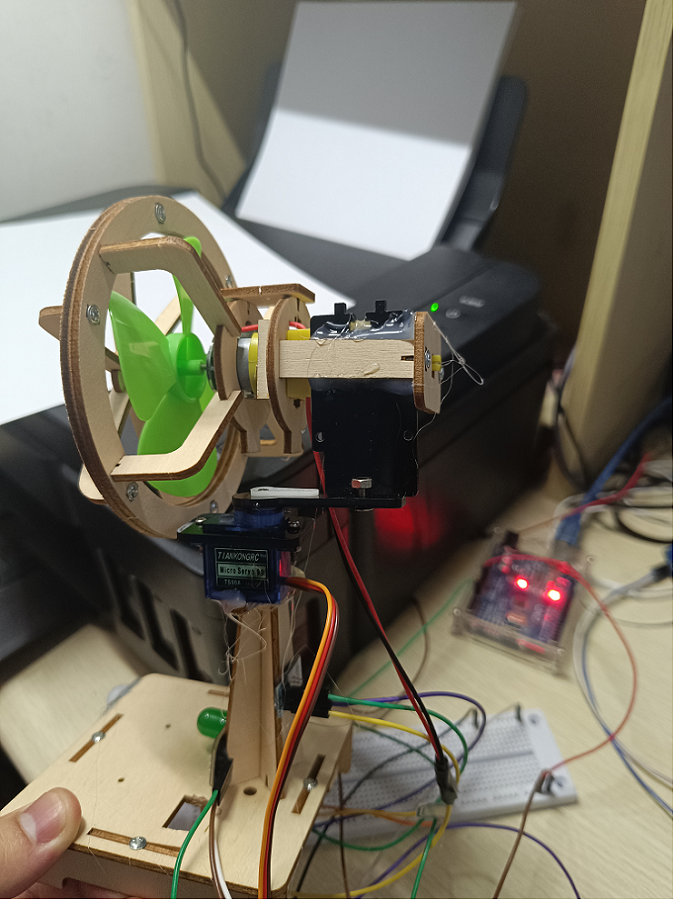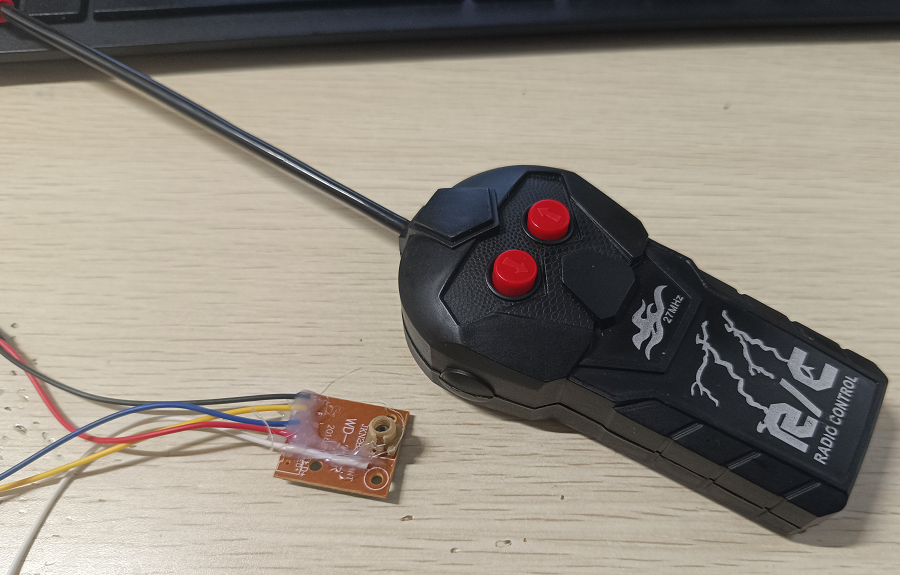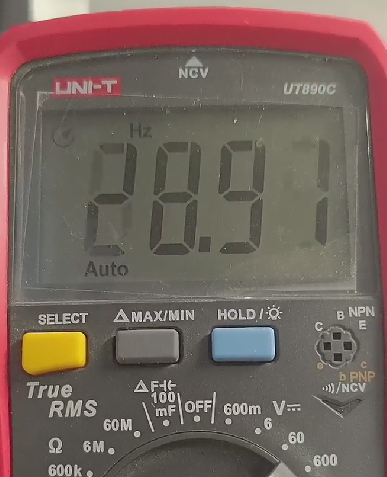## 接线图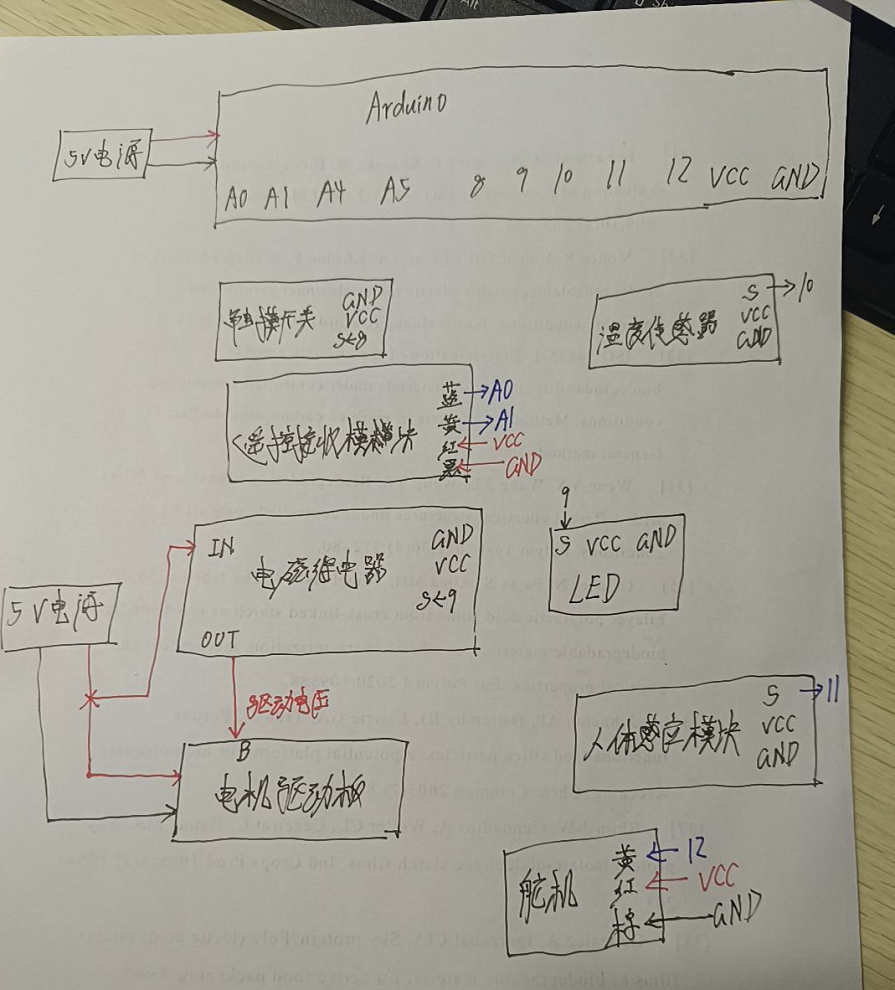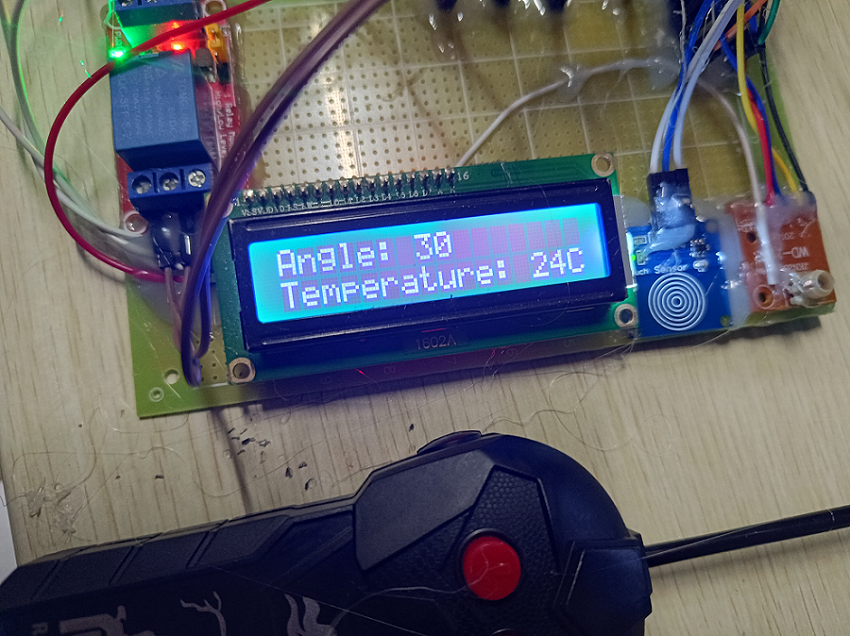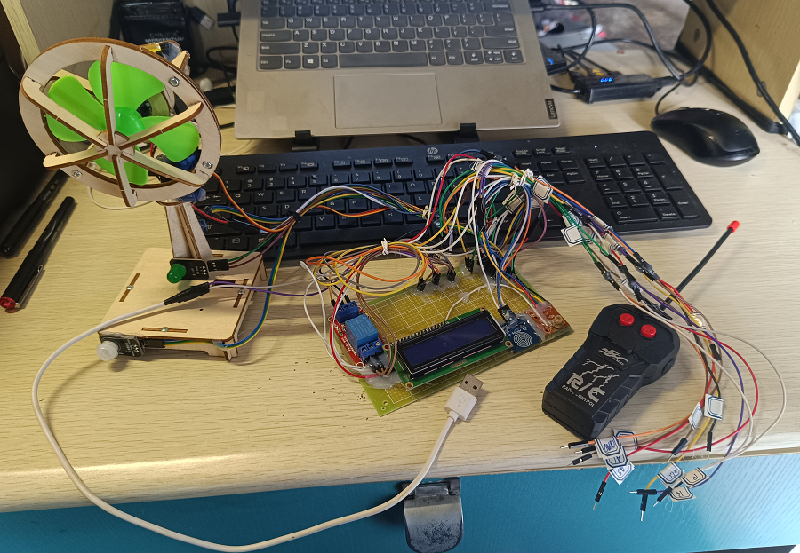## 软件环境

Arduino IDE 2.0.4
Arduino 库：

• LiquidCrystal I2C (by Frank de Brabander) 1.1.2
• OneWire (by Jim Studt…) 2.3.7
• Dallas Temperature (by Miles Burton) 3.9.0
• Servo by Michael Margolis 1.1.8

## 代码

#include <Wire.h>
#include <LiquidCrystal_I2C.h>
#include <OneWire.h>
#include <DallasTemperature.h>
#include <Servo.h>

// 温度传感器
OneWire oneWire(10); // 创建单总线引脚
DallasTemperature temperature(&oneWire); // 温度传感器实例

// LCD 实例
LiquidCrystal_I2C lcd(0x27, 16, 2);

// 舵机实例
Servo servo;

void setup()
{
// LCD 初始化
lcd.init();
lcd.backlight();
lcd.clear();

// 引脚初始化
pinMode(A0, INPUT);
pinMode(A1, INPUT);
pinMode(8, INPUT_PULLUP);
pinMode(9, OUTPUT);
pinMode(11, INPUT_PULLUP);

// 舵机初始化
servo.attach(12);

// 初始化温度传感器
temperature.begin();
}
void loop()
{
static int powerStatus = LOW; // 当前开关状态
static int lastPowerStatus = LOW; // 上一次开关状态
static char lcdString; // 缓冲 LCD 屏显示内容
static float temperatureC = 0; // 温度值
static int angle = 90; // 舵机角度

// 读取温度
temperature.requestTemperatures();
temperatureC = temperature.getTempCByIndex(0);

// 电源触摸开关
lastPowerStatus = powerStatus;

// 舵机左右摆头
if (analogRead(A0) > 950 && powerStatus)
{
angle -= 30;
if (angle < 0)
{
angle = 0;
}
}
else if (analogRead(A1) > 950 && powerStatus)
{
angle += 30;
if (angle > 180)
{
angle = 180;
}
}
servo.write(angle);

// 开机显示标识信息
lcd.clear();
memset(lcdString, 0, sizeof(lcdString));
lcd.setCursor(0, 0);
if (powerStatus && !lastPowerStatus)
{
sprintf(lcdString, "By IYATT-yx");
lcd.print(lcdString);
memset(lcdString, 0, sizeof(lcdString));
sprintf(lcdString, "blog.iyatt.com");
lcd.setCursor(0, 1);
lcd.print(lcdString);
delay(3000);
}

// 显示运行信息
lcd.clear();
lcd.setCursor(0, 0);
memset(lcdString, 0, sizeof(lcdString));
if (powerStatus)
{
sprintf(lcdString, "Angle: %d", angle);
{
digitalWrite(9, HIGH);
}
else
{
digitalWrite(9, LOW);
}
}
else
{
sprintf(lcdString, "Power off");
digitalWrite(9, LOW);
}
lcd.print(lcdString);
lcd.setCursor(0, 1);
memset(lcdString, 0, sizeof(lcdString));
sprintf(lcdString, "Temperature: %dC", (int)temperatureC);
lcd.print(lcdString);
}